﻿ 史上最全的钢材重量计算公式：圆钢、螺纹钢、黄铜、铝..… - 行业新闻 - 新闻资讯 - 山东百炼金属制品IGE赛车机器人

# 关于我们 - 联系我们# 132589909330635-8885206

1米=3.281英尺

1英寸=25。4毫米

1磅=0.4536公斤

1公斤力=9.81牛顿

1磅力=4。45牛顿

1兆帕=145.161磅/英寸

各种钢材理论重量计算公式如下：

 名称（单位） 计算公式 符号意义 圆钢 盘条（kg/m） W= 0.006165×d×d d = 直径mm 直径100mm的圆钢,求m重量。每m 重量= 0。006165×1002=61。65kg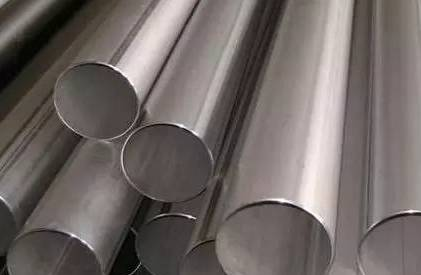螺纹钢（kg/m） W= 0.00617 ×d×d d= 断面直径mm 断面直径为12 mm 的螺纹钢,求每m重量。每m重量=0。00617×12 2=0。89kg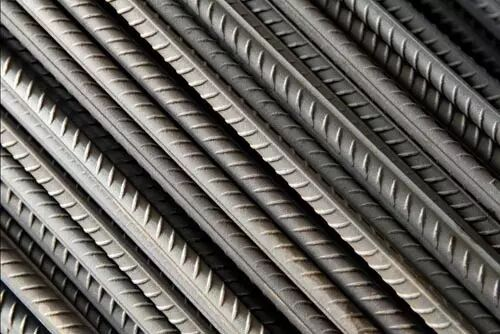方钢（kg/m） W= 0.00785×a×a a= 边宽mm 边宽20 mm 的方钢，求每m 重量。每m重量=0.00785×202=3.14kg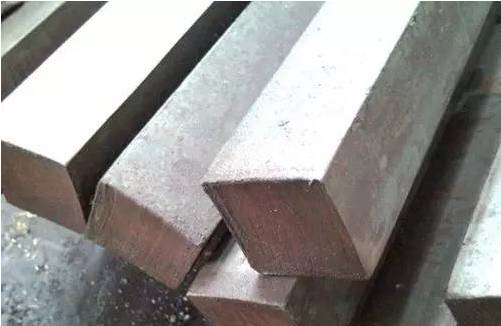扁钢（kg/m） W= 0。00785×b×d b= 边宽mm d= 厚mm 边宽40 mm，厚5mm的扁钢，求每m重量。每m重量= 0.00785×40×5= 1.57kg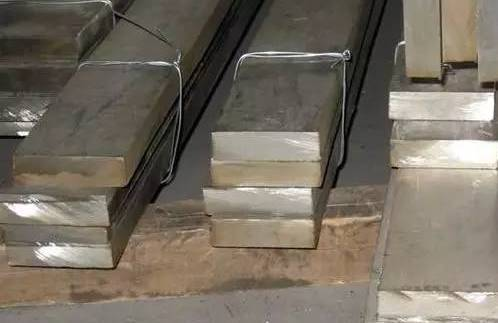六角钢（kg/m） W= 0。006798×s×s s= 对边距离mm 对边距离50 mm的六角钢，求每m 重量。每m 重量= 0.006798×502=17kg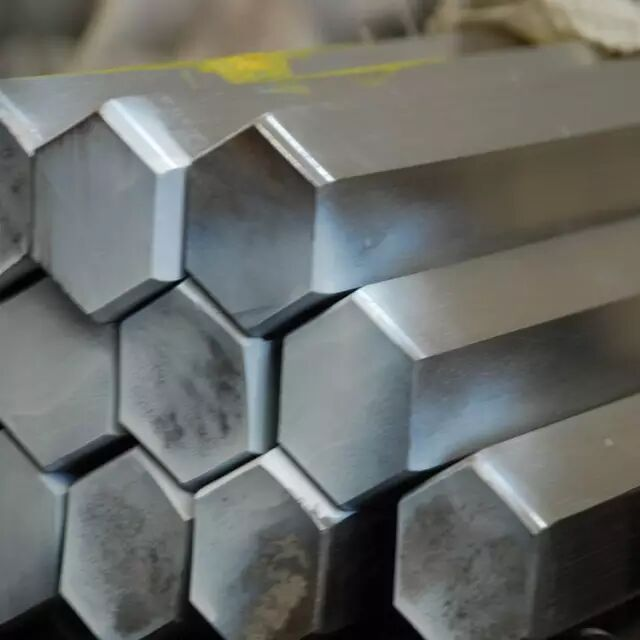八角钢（kg/m） W= 0.0065×s×s s= 对边距离mm 对边距离80mm的八角钢，求每m重量。每m重量=0.0065×802=41.62kg 等边角钢（kg/m） W= 0.00785×[d（2b–d）+0.215（R2– 2r2）] b= 边宽  d= 边厚  R= 内弧半径 r= 端弧半径 求20 mm ×4mm 等边角钢的每m 重量。从冶金产品目录中查出4mm×20 mm 等边角钢的R为3。5,r为1。2,则每m 重量= 0。00785 ×[4×（2×20–4）+0。215×（3。52–2×1。22）]=1。15kg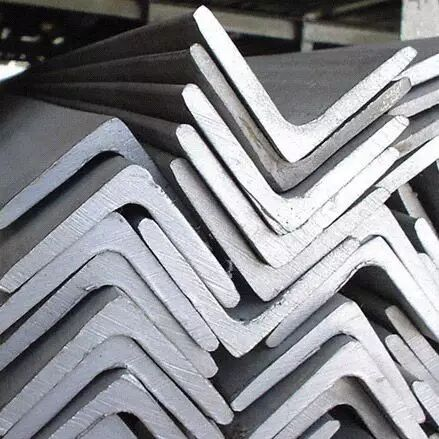不等边角钢（k/m） W=0.00785×[d（B+b – d）+0.215（R2–2r 2）] B= 长边宽  b= 短边宽 d= 边厚  R= 内弧半径 r= 端弧半径 求30 mm×20mm×4mm 不等边角钢的每m 重量。从冶金产品目录中查出30×20×4 不等边角钢的R 为3.5，r为1.2，则每m 重量= 0.00785×[4×（30+20–4）+0.215×（3.52–2×1.22）]=1.46kg 槽钢（kg/m） W=0。00785×[hd+2t （b–d）+0。349（R2–r2）] h= 高  b= 腿长  d= 腰厚 t= 平均腿厚  R= 内弧半径 r= 端弧半径 求80 mm×43mm×5mm 的槽钢的每m 重量。从冶金产品目录中查出该槽钢t为8，R为8，r为4，则每m 重量=0.00785×[80×5+2×8 ×（43–5）+0.349×（82–42）]=8.04kg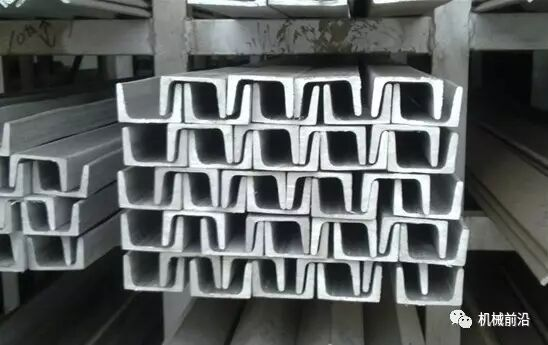工字钢（kg/m） W=0.00785×[hd+2t （b–d）+0.615（R2–r 2）] h= 高  b= 腿长  d= 腰厚  t= 平均腿厚 R= 内弧半径  r= 端弧半径 求250mm×118mm×10mm 的工字钢每m重量。从金属材料手册中查出该工字钢t为13，R为10，r为5，则每m 重量= 0.00785×[250×10+2×13×（118–10）+0.615×（102–52）]=42.03kg 钢板（kg/m2） W= 7.85×d d= 厚 厚度 4mm 的钢板，求每m2 重量。每m2 重量=7.85 ×4=31.4kg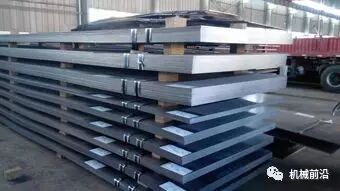钢管（包括无缝钢管及焊接钢管（kg/m） W= 0.02466×S（D– S） D= 外径  S= 壁厚 外径为60 mm 壁厚4mm 的无缝钢管，求m重量。每m重量= 0.02466×4×（60–4）=5.52kg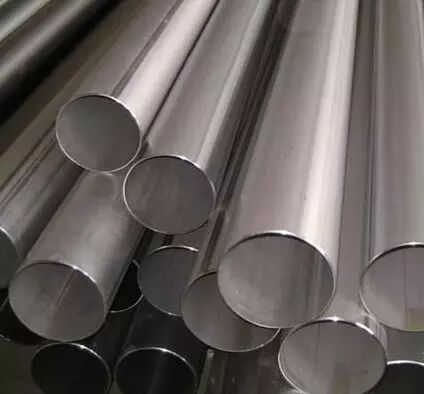重量计算公式 1.铁线：直径mm*直径mm*0.00617*长度mm/1000=重量（kg） 2.铁板：厚度mm*宽度mm*长度mm*0.00785/1000=重量 (kg) 3.圆管：(外径mm-厚度mm)*厚度mm*长度mm*0.02466/1000=重量 (kg) 4.长方管：[(长边+短边)*2/3.1416-厚度]mm*厚度mm*长度mm*0.02466/1000=重量（kg） 5.正方管：（边长*4/3.1416-厚度）mm*厚度mm*长度mm*0.02466/1000=重量 (kg) 【其他有色金属】 黄铜管重量计算公式 公式：(外径-壁厚)×厚×0.0267×长m  例：黄铜管 20mm×1.5mm厚×6m(长) 计算：(20-1。5)×1。5×0。0267×6=4。446kg紫铜管重量计算公式 公式：(外径-壁厚)×厚×0。02796×长m  例：紫铜管 20mm×1.5mm厚×6m(长) 计算：(20-1。5)×1。5×0。02796×6=4。655kg铝花板重量计算公式 公式：长m×宽m×厚mm×2.96  例：铝花板 1m宽×3m长×2.5mm厚 计算：1×3×2。5×2。96=22。2kg 黄铜板：比重8.5 紫铜板：比重8.9 锌板：比重7.2 铅板：比重11.37 计算方式：比重×厚度=每平方的重量 钢板的重量计算方法计算如下： 1）钢管重量(kg)=0。00617*直径*直径*长度；             2）方钢重量(kg)=0.00785*边宽*边宽*长度； 3）六角钢重量(kg)=0.0068*对边宽*对边宽*长度；   4）八角钢重量(kg)=0.0065*对边宽*对边宽*长度； 5）螺纹钢重量(kg)=0.00617*计算直径*计算直径*长度 6）角钢重量(kg)=0.00785*(边宽+边宽-边厚)*边厚*长度； 7）扁钢重量(kg)=0.0075*厚度*边宽*长度；  8）钢管重量(kg)=0.02466*壁厚*(外径-壁厚)*长度； 9）钢板重量(kg)=7.85*厚度*面积；                 10）圆紫铜棒重量(kg)=0.00698*直径*直径*长度； 11)圆黄铜棒重量(kg)=0.00668*直径*直径*长度；       12）圆铝棒重量(kg)=0.0022*直径*直径*长度； 13）方紫铜棒重量(kg)=0.0089*边宽*边宽*长度；       14）方黄铜棒重量(kg)=0.0085*边宽*边宽*边度； 15）方铝棒重量(kg)=0.0028*边宽*边宽*长度；         16）六角紫铜棒重量(kg)=0.0077*对边宽*对边宽*长度； 17）六角黄铜棒重量(kg)=0.00736*边宽*对边宽*长度；  18）六角铝棒重量(kg)=0.00242*对边宽*对边宽*长度； 19）紫铜板重量(kg)=0.0089*厚*宽*长度；             20）黄铜板重量(kg)=0.0085*厚*宽*长度； 21）铝板重量(kg)=0.00171*厚*宽*长度；              22）圆紫铜管重量(kg)=0.028*壁厚*(外径-壁厚)*长度； 23）圆黄铜管重量(kg)=0.0267*壁厚*(外径-壁厚)*长度；    24）圆钢重量(kg)=0.00617*直径*直径*长度； 25）圆铝管重量(kg)=0.00879*壁厚*(外径-壁厚)*长度；    26）无缝管重量(kg)=0.02466*壁厚*(外径-壁厚)*长度； 27）不锈钢管重量(kg/m)=0.02491*壁厚*(外径-壁厚)      28）角钢每米重量=0。00785*(边宽+边宽-边厚)*边厚 （备注：公式中长度单位为米,面积单位为平方米,其余单位均为毫米）